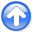Regularization is model stylingNext: Operator approach to missing Up: Missing-data program Previous: Missing-data program

### Matrix approach to missing data

Customarily, we have referred to data by the symbol. Now that we are dividing the data space into two parts, known and unknown (or missing), we refer to this complete space as the model (or map) space.

There are 15 data points in Figures 1-5. Of the 15, 4 are known and 11 are missing. Denote the known byand the missing by. Then, the sequence of missing and known is. Because I cannot printmatrices, please allow me to describe instead a data space of 6 valueswith known values onlyand,arranged as.

Our approach is to minimize the energy in the residual, which is the filtered map (model) space. We state the fitting goalsas:(1)

Rearranging these fitting goals, and bringing the columns multiplying known data values (and) to the left, we get.(2)

Equation (2) is the familiar form of an overdetermined system of equationsthat we could solve foras illustrated earlier by conjugate directions or by a wide variety of well-known methods.

The trouble with this matrix approach is that it is awkward to program the partitioning of the operator into the known and missing parts, particularly if the application of the operator uses arcane techniques, such as those used by the fast-Fourier-transform operator or various numerical approximations to differential, or partial differential, operators that depend on regular data sampling. Even for the modest convolution operator, we already have a library of convolution programs that handle a variety of end effects, and it would be much nicer to use the library as it is rather than recode it for all possible geometrical arrangements of missing data values.Regularization is model stylingNext: Operator approach to missing Up: Missing-data program Previous: Missing-data program

2014-12-03